# RD Sharma Class 10 Ex 5.1 Solutions Chapter 5 Trigonometric Ratios

In this chapter, we provide RD Sharma Class 10 Ex 5.1 Solutions Chapter 5 Trigonometric Ratios for English medium students, Which will very helpful for every student in their exams. Students can download the latest RD Sharma Class 10 Ex 5.1 Solutions Chapter 5 Trigonometric Ratios pdf, Now you will get step by step solution to each question.

# Chapter 5: Trigonometric Ratios Exercise – 5.1

### Question: 1

Find the value of Trigonometric ratios in each of the following provided one of the six trigonometric ratios are given.

(i) sin A = 2/3

(ii) cos A = 4/5

(iii) tan θ = 11/1

(iv) sin θ = 11/15

(v) tan α = 5/12

(vi) sin θ = √3/2

(vii) cos θ = 7/25

(viii) tan θ = 8/15

(ix) cot θ = 12/5

(x)  sec θ = 13/5

(xi)  cosec θ = √10

(xii)  cos θ =12/15

### Solution:

(i) sin A = 2/3

Given: sin A = 2/3  … (1)

By definition,

By Comparing (1) and (2)

We get,

Perpendicular side = 2 and Hypotenuse = 3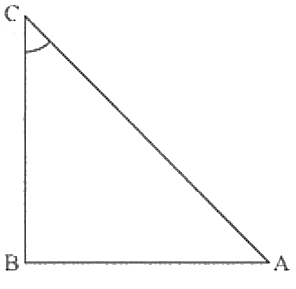Therefore, by Pythagoras theorem,

AC= AB2 + BC2

Substituting the value of perpendicular side (BC) and hypotenuse (AC) and get the base side (AB)

Therefore,

32 = AB2 + 22

AB= 32 – 22

AB2 = 9 – 4

AB= 5

AB = √5

Hence, Base = √5

Therefore,

Therefore,

Therefore,

cot A = √5/2

(ii)  cos A = 4/5

Given: cos A = 4/5  … (1)

By Definition,

By comparing (1) and (2)

We get,

Base = 4 and

Hypotenuse = 5

Therefore,By Pythagoras theorem,

AC= AB+ BC2

Substituting the value of base (AB) and hypotenuse (AC) and get the perpendicular side (BC)

52 = 42+ BC2

BC2 = 52 – 42

BC= 25 – 16

BC2 = 9

BC = 3

Hence, Perpendicular side = 3

Now,

Therefore,

sin A = 3/5

Now, cosec A = 1/(sin A)

Therefore,

cosec A = 1/(sin A)

Therefore,

Therefore,

Therefore,

tan A = 3/4

Now, cot A = 1/(tan A)

Therefore,

By definition,

By Comparing (1) and (2)

We get,

Base = 1 and

Perpendicular side = 5Therefore,

By Pythagoras theorem,

AC2 = AB2 + BC2

Substituting the value of base side (AB) and perpendicular side (BC) and get hypotenuse (AC)

AC2 = 12 + 112

AC2 = 1 + 121

AC= 122

AC = √122

Therefore,

Sin θ = 11√122

Therefore,

Therefore,

Therefore,

By definition,

By Comparing (1) and (2)

We get,

Perpendicular Side = 11 and

Hypotenuse = 15

Therefore,By Pythagoras theorem,

AC2 = AB2 + BC2

Substituting the value of perpendicular side (BC) and hypotenuse (AC) and get the base side (AB)

152 = AB2 +112

AB2 = 15– 112

AB2= 225 – 121

AB= 104

Therefore,

Therefore,

Therefore,

Therefore,

Therefore,

(v)  tan α = 5/12

Given: tan α =  5/12  … (1)

By definition,

By comparing (1) and (2)

We get,

Base = 12 and

Perpendicular side = 5

Therefore,By Pythagoras theorem,

AC2 = AB2 + BC2

Substituting the value of base side (AB) and the perpendicular side (BC) and gte hypotenuse (AC)

AC2 = 122 + 52

AC2 = 144 + 25

AC= 169

AC = 13

Hence Hypotenuse = 13

Therefore,

sin α = 5/13

Therefore,

Therefore,

By definition,

By comparing (1) and (2)

We get,

Perpendicular side = √3

Hypotenuse = 2

Therefore,By Pythagoras theorem,

AC2 = AB2 + BC2

Substituting the value of perpendicular side (BC) and hypotenuse (AC) and get the base side (AB)

AB2 = 4 – 3

AB2 = 1

AB = 1

Hence Base = 1

Therefore,

cos θ = 1/2

Now, cosec θ = 1/sin θ

Therefore,

Therefore,

Therefore,

Therefore,

(vii) cos θ = 7/25Given:  cos θ = 7/25        … (1)

By definition,

By comparing (1) and (2)

We get,

Base = 7 and

Hypotenuse = 25

Therefore

By Pythagoras theorem,

AC = AB2 + BC2

Substituting the value of base side (AB) and hypotenuse (AC) and get the perpendicular side (BC)

252 = 7+ BC2

BC2 = 252 – 72

BC2 = 625 – 49

BC = 576

BC = √576

BC = 24

Hence, Perpendicular side = 24

Therefore,

Sin θ = 24/25

Now, cosec θ = 1/sin θ

Therefore,

Therefore,

Therefore,

Therefore,

By definition,

By comparing (1) and (2)

We get,

Base = 15 and Perpendicular side = 8Therefore,

By Pythagoras theorem,

AC2= 152 + 82

AC2= 225 + 64

AC2 = 289

AC = √289

AC = 17

Hence, Hypotenuse = 17

Therefore,

sin θ = 8/17

Now, cosec θ = 1/sin θ

Therefore,

Therefore,

cos θ =15/17

Now, sec θ = 1/cos θ

Therefore,

Therefore,

(ix) cot θ = 12/5

Given: cot θ = 12/5  … (1)

By definition,

By comparing (1) and (2)

We get,

Base = 12 and

Perpendicular side = 5

Therefore,By Pythagoras theorem,

AC= AB2 + BC2

Substituting the value of base side (AB) and perpendicular side (BC) and get the hypotenuse (AC)

AC2 = 122 + 52

AC= 144 + 25

AC2 = 169

AC = √169

AC = 13

Hence, Hypotenuse = 13

Therefore,

sin θ = 5/13

Now, cosec θ = 1/sin θ

Therefore,

Therefore,

cos θ = 12/13

Now, sec θ = 1/cos θ

Therefore,

Therefore,

(x) sec θ = 13/5

Given: sec θ = 13/5… (1)

By definition,

By comparing (1) and (2)

We get,

Base = 5

Hypotenuse = 13

Therefore,By Pythagoras theorem,

Substituting the value of base side (AB) and hypotenuse (AC) and get the perpendicular side (BC)

132 = 52 + BC2

BC2 = 13– 52

BC= 169 – 25

BC= 144

BC = √144

BC = 12

Hence, Perpendicular side = 12

Therefore,

sin θ = 12/13

Now, cosec θ = 1/sin θ

Therefore,

Therefore,

Therefore,

tan θ = 12/5

Now, cot θ = 1/tan θ

Therefore,

(xi) cosec θ = √10

Given:  cosec θ = √10/1 … (1)

By definition

By comparing (1) and (2)

We get,

Perpendicular side = 1 and

Hypotenuse = √10

Therefore,By Pythagoras theorem,

AC= AB2 + BC2

Substituting the value of perpendicular side (BC) and hypotenuse (AC) and get the base side (AB)

AB= 10 – 1

AB = √9

AB = 3

Hence, Base side = 3

Therefore,

Therefore,

Therefore,

cot θ = 3

(xii)  cos θ = 12/15

Given: cos θ = 12/15 … (1)

By definition,

By comparing (1) and (2)

We get,

Base = 12 and Hypotenuse = 15

Therefore,By Pythagoras theorem,

AC2 = AB+ BC2

Substituting the value of base side (AB) and hypotenuse (AC) and get the perpendicular side (BC)

152 = 122 + BC2

BC2 = 152 – 122

BC2 = 225 – 144

BC 2= 81

BC = √81

BC = 9

Hence, Perpendicular side = 9

Therefore,

Therefore,

Therefore,

Therefore,

Therefore,

### Question: 2

In a ΔABC, right angled at B, AB – 24 cm, BC = 7cm, Determine

(i) sin A, cos A

(ii) sin C, cos C

### Solution:

(i) The given triangle is below: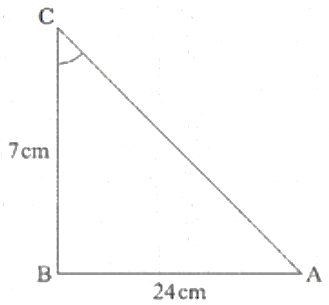Given: In ΔABC, AB = 24 cm

BC = 7cm

∠ABC = 90°

To find: sin A, cos A

In this problem, Hypotenuse side is unknown

Hence we first find hypotenuse side by Pythagoras theorem

By Pythagoras theorem,

We get,

AC2 = AB2 + BC2

AC2 = 242 + 72

AC2 = 576 + 49

AC= 625

AC = √625

AC = 25

Hypotenuse = 25

By definition,

By definition,

(ii)  The given triangle is below:Given: In Δ ABC, AB = 24 cm

BC = 7cm

∠ABC = 90°

To find: sin C, cos C

In this problem, Hypotenuse side is unknown

Hence we first find hypotenuse side by Pythagoras theorem

By Pythagoras theorem,

We get,

AC2 = AB2 + BC2

AC2 = 242 + 72

AC2 = 576 + 49

AC= 625

AC = √625

AC = 25

Hypotenuse = 25

By definition,

By definition,

By definition,

### Question: 3

In the below figure, find tan P and cot R. Is tan P = cot R? To find, tan P, cot R

### Solution:

In the given right angled ΔPQR, length of side OR is unknown

Therefore, by applying Pythagoras theorem in ΔPQR

We get,

PR2 = PQ2 + QR2

Substituting the length of given side PR and PQ in the above equation

13= 122 + QR2

QR2 = 132 – 122

QR2 = 169 – 144

QR2= 25

QR =√25

By definition, we know that,

Also, by definition, we know that

Comparing equation (1) ad (2), we come to know that that R.H.S of both the equation are equal.

Therefore, L.H.S of both equations is also equal

tan P = cot R

### Question: 4

If sin A = 9/41, Compute cos A and tan A.

### Solution:

Given: sin A = 9/41  … (1)

To find: cos A, tan A

By definition,

By comparing (1) and (2)

We get,

Perpendicular side = 9 and Hypotenuse = 41

Now using the perpendicular side and hypotenuse we can construct ΔABC as shown figure.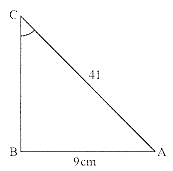Length of side AB is unknown is right angled ΔABC,

To find the length of side AB, we use Pythagoras theorem,

Therefore, by applying Pythagoras theorem in ΔABC,

We get,

AC2 = AB2 + BC2

412 = AB2 + 92

AB2 = 412 – 92

AB2 = 168 – 81

AB= 1600

AB = √1600

AB = 40

Hence, length of side AB = 40

Now

By definition,

Now,

By definition,

### Question: 5

Given 15 cot A = 8, find sin A and sec A.

### Solution:

Given: 15 cot A = 8

To find: sin A, sec A

Since 15 cot A =8

By taking 15 on R.H.S

We get,

Since 15 cot A = 8

By taking 15 on R.H.S

We get,

cot A = 8/15

By definition,

cot A = 1/(tan A)

Hence,

Comparing equation (1) and (2)

We get,

Base side adjacent to ∠A = 8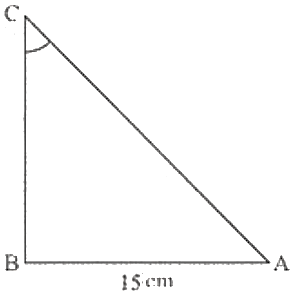Perpendicular side opposite to ∠A = 15

ΔABC can be drawn below using above information

Hypotenuse side is unknown.

Therefore, we find side AC of ΔABC by Pythagoras theorem.

So, by applying Pythagoras theorem to ΔABC

We get,

AC2 = AB2 +BC2

Substituting values of sides from the above figure

AC2 = 82 + 152

AC2 = 64 + 225

AC2 = 289

AC = √289

AC = 17

Therefore, hypotenuse =17

Now by definition,

Substituting values of sides from the above figure

Sin A = 15/17

By definition,

sec A = 1/cos A

Hence,

Substituting values of sides from the above figure

Sec A = 17/8

sin A =15/17, sec A = 17/8

### Question: 6

In ΔPQR, right angled at Q, PQ = 4cm and RQ = 3 cm .Find the value of sin P, sin R, sec P and sec R.

### Solution:

Given: ΔPQR is right angled at vertex Q.

PQ = 4cm

RQ = 3cm

To find,

sin P, sin R, sec P, sec R

Given ΔPQR is as shown figure.

Hypotenuse side PR is unknown.

Therefore, we find side PR of ΔPQR by Pythagoras theorem

By applying Pythagoras theorem to ΔPQR

We get,

PR2 = PQ2 + RQ2

Substituting values of sides from the above figure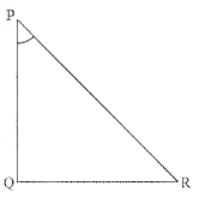PR2 = 42 +32

PR2 = 16 + 9

PR2 = 25

PR = √25

PR = 5

Hence, Hypotenuse =5

Now by definition,

sin P = RQ/PR

Substituting values of sides from the above figure

sin P = 3/5

Now by definition,

sin R = PQ/PR

Substituting the values of sides from above figure

sin R = 4/5

By definition,

sec P = 1/cos P

Substituting values of sides from the above figure

sec P = PR/PQ

sec P = 5/4

By definition,

sec R = 1/cos R

Substituting values of sides from the above figure

sec R = PR/RQ

sec R = 5/3

sin P = 3/5,

sin R = 4/5,

sec P = 5/4,

sec R = 5/3

### Question: 7

If cot θ = 7/8, evaluate

(ii)  cotθ cot2θ

### Solution:

Given: cot θ = 7/8

We know the following formula

(a + b)(a – b) = a2 – b2

By applying the above formula in the numerator of equation (1)

We get,

(1 + sin θ) × (1 – sin θ) = 1 – sin2θ …. (2) (Where, a = 1 and b = sin θ)

Similarly,

By applying formula (a + b) (a – b) = a2 – b2 in the denominator of equation (1).

We get,

(1 + cos θ)(1 – cos θ) = 1 – cosθ (1 + cos θ)(1 – cos θ) = 1 – cos2θ … (Where a = 1 and b = cos θ cos θ

Substituting the value of numerator and denominator of equation (1) from equation (2), equation (3).

Therefore,

Since,

cos2θ + sin2 θ = 1 cos2 θ + sin2 θ = 1

Therefore,

cos2 θ = 1 – sin2Θcos2 θ =1 – sin2 θ

Also, sin2 θ = 1 – cos2 θ sin2 θ = 1 – cos2 θ

Putting the value of 1 – sin2 θ and 1 – cos2 θ in equation (4)

We get,

We know that,

Since, it is given that cot θ = 7/8

Therefore,

(ii) Given: cot θ = 7/8

To evaluate: cot2 θ

cot θ  =7/8

Squaring on both sides,

We get,

49/64

### Question: 8

If 3 cot A = 43 cot A = 4, check whether

### Solution:

Given: 3 cot A = 4

To check whether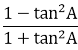cos2A –sin2A or not.

3 cot A = 4

Dividing by 3 on both sides,

We get,

cot A = 4/3  … (1)

By definition,

cot A = 1/tan A

Therefore,

Comparing (1) and (2)

We get,

Base side adjacent to ∠A = 4

Perpendicular side opposite to ∠A = 3

Hence ΔABC is as shown in figure.In ΔABC , Hypotenuse is unknown

Hence, it can be found by using Pythagoras theorem

Therefore by applying Pythagoras theorem in ΔABC

We get

AC= AB2 + BC2

Substituting the values of sides from the above figure

AC2 = 42 + 32

AC2 = 16 + 9

AC2 = 25

AC = √25

AC = 5

Hence, hypotenuse = 5

To check whethercos2A – sin2A or not.

We get thee values of tan A, cos A, sin A

By definition,

tan A =1/(cot A)

Substituting the value of cot A from equation (1)

We get,

tan A = 1/4

tan A = 3/4  …. (3)

Now by definition,

Substituting the values of sides from the above figure

Now we first take L.H.S of equation

Substituting value of tan A from equation (3)

We get,

Taking L.C.M on both numerator and denominator

We get,

Now we take R.H.S of equation whether

R.H.S = cos2A – sin2A

Substituting value of sin A and cos A from equation (4) and (5)

We get,

Comparing (6) and (7)

We get.

### Question: 9

If tan θ = a/b, find the value of### Solution:

Given: tan θ = a/b  … (1)

Now, we know that

Therefore equation (1) become as follows

Now, by applying invertendo

We get,

Now by applying Componendo – dividendo

We get,

Therefore,

### Question: 10

If 3 tan θ = 4, find the value of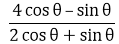### Solution:

Given: If 3 tan θ = 4/3 tan θ = 4

Therefore,

tan θ = 4/3  … (1)

Now, we know that

Therefore equation (1) becomes

Now, by applying Invertendo to equation (2)

We get,

Now, multiplying by 4 on both sides

We get

Therefore

Now, multiplying by 2 on both sides of equation (3)

We get,

Now by applying componendo in above equation

We get,

Therefore,

Therefore, on L.H.S sin θ sin θ cancels and we get,

Therefore,

4 cos θ – sin θ = 44 cos θ – sin θ = 4

### Question: 11

If 3 cot θ = 2, find the value of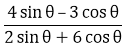### Solution:

Given: 3 cot θ = 2

Therefore,

Now, we know that

Therefore equation (1) becomes

Now, by applying invertendo to equation (2)

Now, multiplying by 4/3 on both sides,

We get,

Therefore, 3 cancels out on R.H.S and

We get,

Now by applying invertendo dividendo in above equation

We get,

Now, multiplying by 2/6 on both sides of equation (3)

We get,

Therefore, 2 cancels out on R.H.S and

We get,

Now by applying componendo in above equation

We get,

Now, by dividing equation (4) by (5)

We get,

Therefore,

Therefore, on L.H.S (3 sin θ) cancels out and we get,

Now, by taking 2 in the numerator of L.H.S on the R.H.S

We get,

Therefore, 2 cancels out on R.H.S and

We get,

### Question: 12

If tan θ = a/b, prove that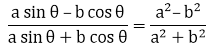### Solution:

Given:

Now, we know that

Therefore equation (1) becomes

Now, by multiplying by a/b on both sides of equation (2)

We get,

Therefore,

Now by applying dividendo in above equation (3)

We get,

Now by applying componendo in equation (4)

We get,

Now, by dividing equation (4) by equation (5)

We get,

Therefore,

Therefore, b cos θ and bcancels on L.H.S and R.H.S respectively

Hence, it is proved that

### Question: 13

If sec θ = 13/5, show that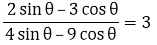### Solution:

Given: sec θ = 13/5

To show that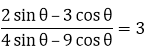Now, we know that

Therefore,

Therefore,

Now, we know that

Now, by comparing equation (1) and (2)

We get,

Base side adjacent to ∠θ = 5

And

Hypotenuse =13

Therefore from above figure

Base side BC = 5

Hypotenuse AC = 13

Side AB is unknown. It can be determined by using Pythagoras theorem

Therefore by applying Pythagoras theorem

We get,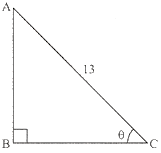AC2 = AB2 + BC2

Therefore by substituting the values of known sides

We get,

132 = AB2 + 52

Therefore,

AB2= 132 – 52

AB2= 169 – 25

AB2 = 144

AB = √144

Therefore,

AB = 12 …. (3)

Now, we know that

sin θ = AB/AC

sin θ = 12/13  … (4)

Now L.H.S of the equation to be proved is as follows

Substituting the value cos θ of sin θ and from equation (1) and (4) respectively

We get,

Therefore,

L.H.S = 3

Hence proved that,

### Question: 14

If cos θ = 12/13, show that sin θ(1 – tan θ) = 35/156

### Solution:

To show that

Now we know that

Therefore, by comparing equation (1) and (2)

We get,

Base side adjacent to ∠θ = 12

And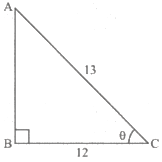Hypotenuse = 13

Therefore from above figure

Base side BC = 12

Hypotenuse AC = 13

Side AB is unknown and it can be determined by using Pythagoras theorem

Therefore by applying Pythagoras theorem

We get,

AC2= AB2 + BC2

Therefore by substituting the values of known sides

We get,

132= AB2 + 122

Therefore,

AB2= 13– 122

AB2= 169 – 144

AB = 25

AB = √25

AB = 5 …. (3)

Now, we know that

Now from figure (a)

We get,

sin θ = AB/AC

Therefore,

sin θ = 5/12… (5)

Now L.H.S of the equation to be proved is as follows

L.H.S of the equation to be proved is as follows

L.H.S = sin θ (1 – tan θ] …. (6)

Substituting the value of sin θ and tan θ from equation (4) and (5)

We get,

Taking L.C.M inside the bracket

We get,

Therefore,

Now by opening the bracket and simplifying

We get,

From equation (6) and (7),it can be shown that

### Solution:

Now, we know that

Therefore,

Therefore,

Comparing Equation (1) and (2)

We get.

Base side adjacent to ∠θ = 1

Perpendicular side opposite to ∠θ = √3

Therefore, triangle representing angle √3 is as shown below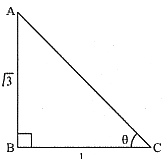Therefore, by substituting the values of known sides

We get,

Therefore,

AC2 = 3 + 1

AC= 4

AC = √4

Therefore,

AC = 2 …   (3)

Now, we know that

Now from figure (a)

Therefore from figure (a) and equation (3),

Now we know that

Now from figure (a)

We get, BC/AC

Therefore from figure (a) and equation (3),

Now, L.H.S of the equation to be proved is as follows

Substituting the value of from equation (4) and (5)

We get,

Now by taking L.C.M in numerator as well as denominator

We get,

Therefore,

Therefore,

### Solution:

To show that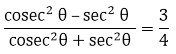Now, we know that

Therefore,

Comparing equation (1) and (2)

We get.

Perpendicular side opposite to ∠θ = 1

Base side adjacent to ∠θ = √7

Therefore, Triangle representing ∠ θ is shown figure.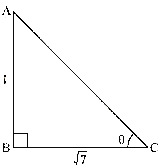Hypotenuse AC is unknown and it can be found by using Pythagoras theorem

Therefore by applying Pythagoras theorem

We get,

AC= AB2 + BC2

Therefore by substituting the values of known sides

We get,

Therefore,

AC 2 = 1 +7

AC2 = 8

AC = √8

Therefore,

Now we know that

Now, we know that

Therefore, from equation (4)

We get,

Now, we know that

Now from figure (a)

We get,

cos θ = BC/AC

Therefore from figure (a) and equation (3)

Now we know that

Therefore, from equation (6)

We get,

Now, L.H.S of the equation to be proved is as follows

Substituting the value of cosec θ and sec θ from equation (6) and (7)

We get,

Therefore,

Therefore,

L.H.S = 48/64

L.H.S = 3/4 = R.H.S

Therefore,

Hence proved that

### Question: 17

If sec θ = 5/4, find the value of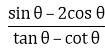### Solution:

To find the value of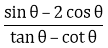Now we know that

Therefore,

Therefore from equation (1)

Also, we know that cos2θ + sin2θ = 1

Therefore,

Substituting the value of cos θ cos θ from equation (2)

We get,

Therefore,

Also, we know that

sec2θ  =1 + tanθ secθ = 1 + tanθ

Therefore,

Therefore,

tan θ = 3/4  … (4)

Also, cot θ = 1/tan θ

Therefore from equation (4)

We get,

cot θ = 4/3  … (5)

Substituting the value of cos θ, cot θ and tan θ from the equation (2), (3), (4) and (5) respectively in the expression below

We get,

= 12/7

Therefore,

### Question: 18

If sin θ = 12/13, find the value of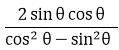### Solution:

To  find the value ofNow, we know the following trigonometric identity

cosecθ = 1 + tan2θ

Therefore, by substituting the value of tan θ from equation (1)

We get,

By taking L.C.M on the R.H.S

We get,

Therefore

Therefore

Now, we know that

Therefore

Now, we know the following trigonometric identity

cos2θ + sin2θ = 1

Therefore,

cos2θ = 1 – sin2θ

Now by substituting the value of sin θ from equation (3)

We get,

Therefore, by taking L.C.M on R.H.S

We get,

Now, by taking square root on both sides

We get,

Therefore,

Substituting the value of sin θ and cos θ from equation (3) and (4) respectively in the equation below

Therefore,

Therefore,

### Question: 19

If cos θ = 3/5, find the value of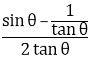### Solution:

To find the value ofNow we know the following trigonometric identity

cos2θ + sin2θ = 1

Therefore by substituting the value of cos θ from equation (1)

We get,

Therefore,

Therefore by taking square root on both sides

We get,

Now, we know that

Therefore by substituting the value of sin θ and cos θ from equation (2) and (1) respectively

We get,

Now, by substituting the value of sin θ and of tan θ from equation (2) and equation (4) respectively in the expression below

We get,

Therefore,

### Question: 20

If sin θ = 3/5, find the value of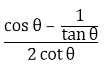### Solution:

Given:

Now, we know the following trigonometric identity

cos2θ + sin2θ = 1

Therefore by substituting the value of cos θ from equation (1)

We get,

Therefore,

Now by taking L.C.M

We get,

Therefore, by taking square roots on both sides

We get,

cos θ = 4/5

Therefore,

cos θ = 4/5  … (2)

Now we know that

tan θ = sin θ/cos θ

Therefore by substituting the value of sin θ and cos θ from equation (1) and (2) respectively

We get,

Also, we know that

Therefore from equation (3)

We get,

Now by substituting the value of cos θ, tan θ and cot θ from equation (2), (3) and (4) respectively from the expression below

Therefore,

### Question: 21

If tan θ = 24/7, find that sin θ + cos θ

### Solution:

Given: tan θ =24/7  … (1)

To find, Sin θ + cos θ

Now we know that tan θ is defined as follows

Now by comparing equation (1) and (2)

We get,

Perpendicular side opposite to ∠θ = 24

Base side adjacent to ∠θ = 7

Therefore triangle representing ∠θ is as shown figure.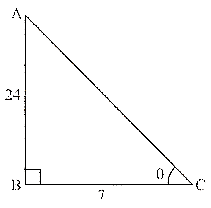Side AC is unknown and can be found by using Pythagoras theorem

Therefore,

AC= AB2 + BC2

Now by substituting the value of unknown sides from figure

We get,

AC2= 242 +72

AC = 576 + 49

AC = 625

Now by taking square root on both sides,

We get,

AC = 25

Therefore,

Hypotenuse side AC = 25 …. (3)

Now we know sin θ is defined as follows

Therefore from figure (a) and equation (3)

We get,

Now we know that cos θ is defined as follows

Therefore by substituting the value of sin θ and cos θ from equation (4) and (5) respectively, we get

### Question: 21

If sin θ = a/b, find sec θ + tan θ in terms of a and b.

### Solution:

Given:

sin θ = a/b  … (1)

To find:  sec θ + tan θ

Now we know, sin θ is defined as follows

Now by comparing equation (1) and (2)

We get,

Perpendicular side opposite to ∠θ = a

Hypotenuse = b

Therefore triangle representing ∠θ is as shown figure.

Hence side BC is unknown

Now we find BC by applying Pythagoras theorem to right angled ΔABC

Therefore,

AC2 = AB2 + BC2

Now by substituting the value of sides AB and AC from figure (a)

We get,

b2 = a2 + BC2

Therefore,

BC2 = b2 – a2

Now by taking square root on both sides

We get,

Therefore,

Now we know cos θ is defined as follows

Therefore from figure (a) and equation (3)

We get,

Now we know,

Therefore,

Now we know,

Now by substituting the values from equation (1) and (3)

We get,

Therefore,

Now we need to find sec θ + tan θ

Now by substituting the values of sec θ and tan θ from equation (5) and (6) respectively

We get,

We get,

Now by substituting the value in above expression

We get,

Now,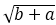present in the numerator as well as denominator of above denominator of above expression gets cancels we get,

Square root is present in the numerator as well as denominator of above expression. Therefore we can place both numerator and denominator under a common square root sign

Therefore,

### Question: 23

If 8 tan A = 15, find sin A – cos A

### Solution:

Given:

8 tan A = 15

Therefore,

To find: sin A – cos A

Now we know tan A is defined as follows

Now by comparing equation (1) and (2)

We get

Perpendicular side opposite to ∠A = 15

Base side adjacent to ∠A = 8

Therefore triangle representing angle A is as shown figure.

Side AC = is unknown and can be found by using Pythagoras theorem

Therefore,

AC= AB2 + BC2

Now by substituting the value of known sides from figure (a)

We get,

AC= 152 + 82

AC2 = 225 + 64

AC = 289

Now by taking square root on both sides

We get,

AC = √289

AC = 17

Therefore Hypotenuse side AC = 17 … (3)

Now we know, sin A is defined as follows

Therefore from figure (a) and equation (3)

We get,

Now we know, cos A is defined as follows

Therefore from figure (a) and equation (3)

We get,

Now we find the value of expression sin A – cos A

Therefore by substituting the value the value of sin A and cos A from equation (4) and (5) respectively, we get,

### Question: 24

If tan θ = 20/21, show that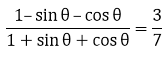### Solution:

Given: tan θ = 20/21

Now we know that

Therefore,

tan θ = 20/21

Side AC be the hypotenuse and can be found by applying Pythagoras theorem

Therefore,

AC= AB2 + BC2

AC2= 212 + 202

AC2 = 441 + 400

AC2 = 841

Now by taking square root on both sides

We get,

AC = √841

AC = 29

Therefore Hypotenuse side AC = 29

Now we know, sin θ is defined as follows,

Therefore from figure and above equation

We get,

Now we know cos θ is defined as follows

Therefore from figure and above equation

We get,

Now we need to find the value of expression

Therefore by substituting the value of sin θ and cos θ from above equations, we get

Therefore after evaluating we get,

Hence,

### Question: 25

If cosec A = 2, find### Solution:

Given: cosec A = 2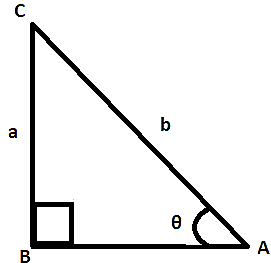Here BC is the adjacent side,

By applying Pythagoras theorem,

AC= AB2 + BC2

4 = 1 + BC2

BC= 3

BC = √3

Now we know that

Substitute all the values of sin A, cos A and tan A from the equations (1), (2) and (3) respectively

We get.

= 2

Hence,

### Question: 26

If ∠A and ∠B are acute angles such that cos A =cos B, then show that ∠A = ∠B

### Solution:

Given:

∠A and ∠B are acute angles

cos A = cos B such that ∠A = ∠B

Let us consider right angled triangle ACB

Now since cos A = cos B

Therefore

Now observe that denominator of above equality is same that is AB

Therefore AC = BC

We know that when two sides of triangle are equal, then opposite of the sides are also

Equal.

Therefore

We can say that

Angle opposite to side AC = angle opposite to side BC

Therefore,

∠B = ∠A

Hence, ∠A = ∠B

### Question: 27

In a ΔABC, right angled triangle at A, if tan C = √3, find the value of sin B cos C + cos B sin C.

### Solution:

Given: ΔABC

To find: sin B cos C + cos B sin C

The given a ΔABC is as shown in figureSide BC is unknown and can be found using Pythagoras theorem,

Therefore,

BC= AB2 + AC2

BC= 3 +1

BC2 = 4

Now by taking square root on both sides

We get,

BC = √4

BC = 2

Therefore Hypotenuse side BC = 2 … (1)

Now,

Therefore,

Now by substituting the values from equation (1) and figure

We get,

Now,

Therefore,

Now substituting the value from equation

Similarly

Now by definition,

So by evaluating

Now, by substituting the value of sin B, cos B, sin C and cos C from equation (2), (3), (4) and (5) respectively in sin B cos C + cos B sin C

Sin B cos C + cos B sin C

= 1

Hence,

sin B cos C + cos B sin C = 1

### Question: 28

(i) The value of tan A is always less than 1.

(ii) sec A = 12/5 for some value of ∠A.

(iii) cos A is the abbreviation used for the cosecant of ∠A.

(iv) sin θ = 4/3 for some angle θ.

### Solution:

(i) tan A < 1

Value of tan A at 45° i.e… tan 45 = 1

As value as A increases to 90°

Tan A becomes infinite

So given statement is false.

(ii) sec A = 12/5 for some value of angle if

M-I

sec A = 2.4

sec A > 1

So given statements is true.

M- II

For sec A = 12/5 we get adjacent side = 13

Subtending 9i at B.

So, given statement is true.

(iii) Cos A is the abbreviation used for cosecant of angle A.

The given statement is false.

As such cos A is the abbreviation used for cos of angle A, not as cosecant of angle A.

(iv) cot A is the product of cot A and A

Given statement is false? cot A is a co-tangent of angle A and co-tangent of angle

(v) sin θ = 4/3 for some angle θ.

Given statement is false

Since value of sin θ is less than (or) equal to one.

Here value of sin θ exceeds one,

So given statement is false.

### Solution:

As shown in figure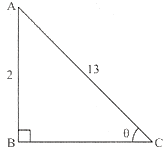Here BC is the adjacent side,

By applying Pythagoras theorem,

AC= AB+ BC2

169 = 144 + BC2

BC= 169 – 144

BC= 25

BC = 5

Now we know that,

We also know that,

tan θ = sin θ/cos θ

Therefore, substituting the value of sin θ and cos θ from above equations

We get,

tan θ = 12/5

Now substitute all the values of sin θ, cos θ and tan θ from above equations in

We get,

Therefore by further simplifying we get,

Therefore,

Hence,

### Question: 30

If cos θ = 5/13, find the value of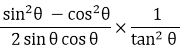### Solution:

Given: If cos θ = 5/13   ….. (1)

To find: The value of expressionNow we know that

Now when we compare equation (1) and (2)

We get,

Base side adjacent to ∠θ = 5

Hypotenuse = 13

Therefore, Triangle representing ∠θ is as shown figure.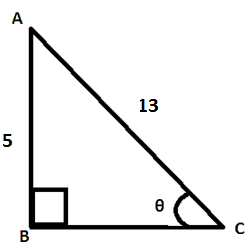Perpendicular side AB is unknown and it can be found by using Pythagoras theorem

Therefore by applying Pythagoras theorem

We get,

AC2= AB2 + BC2

Therefore by substituting the values of known sides,

AB2 = 132 – 52

AB2= 169 -25

AB2 = 144

AB = 12 …. (3)

Now we know from figure and equation,

Now we know that,

Now w substitute all the values from equation (1), (4) and (5) in the expression below,

Therefore

We get,

Therefore by further simplifying we get,

Therefore,

Hence,

### Solution:

Given: sec A = 17/8

Now we know that

Now, by substituting the value of sec A

We get,

Now we also know that,

sin2A + cos2A = 1

Therefore

sin2A = 1 – cos2A

Now by taking square root on both sides,

We get,

sin A = 15/17

We also know that,

Now by substituting the value of all the terms,

We get,

tan A = 15/8

Now from the expression of above equation which we want to prove:

Now by substituting the value of cos A ad sin A from equation (3) and (4)

We get,

From expression

Now by substituting the value of tan A from above equation

We get,

Therefore,

We can see that,

If sin θ = 3/4,

Prove that

### Solution:

To prove:

By definition,

By comparing (1) and (3)

We get,

Perpendicular side = 3 and

Hypotenuse = 4

Side BC is unknown.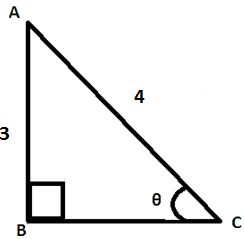So we find BC by applying Pythagoras theorem to right angled ΔABC

Hence,

AC2 = AB2 + BC2

Now we substitute the value of perpendicular side (AB) and hypotenuse (AC) and get the base side (BC)

Therefore,

4= 32 + BC2

BC2 = 16 – 9

BC= 7

BC = √7

Hence, Base side BC = √7 … (3)

Now cos A = BC/AC

√7/4    … (4)

Now, cosec A = 1/(sin A)

Therefore, from fig and equation (1)

cosec A = 4/3  … (5)

Now, similarly

Sec A = 4/√7  … (6)

Further we also know that

Therefore by substituting the values from equation (1) and (4),

We get,

Now by substituting the value of cosec A, sec A and cot A from the equations (5), (6), and (7) in the L.H.S of expression (2)

Hence it is proved that,

### Question: 33

If sec A = 17/8,

Verify that### Solution:

Given: sec A = 17/8     … (1)

To verify:

Now we know that

sec A = 1/(cos A)

Therefore,

cos A = 1/(sec A)

We get,

cos A = 8/17  … (3)

Similarly we can also get,

sin A = 15/17  …. (4)

An also we know that

tan A = (sin A)/(cos A)

tan A = 15/8  … (5)

Now from the expression of equation (2)

L.H.S: Missing close

Now by substituting the value of cos A and sin A from equation (3) and (4)

We get,

Now by substituting the value of tan A from equation (5)

We get,

Now by comparing equation (6) and (7)

We get,

### Question: 34

If cot θ = 3/4, prove that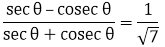### Solution:

Given: cot θ = 3/4

Prove that:Now we know thatHere AC is the hypotenuse and we can find that by applying Pythagoras theorem

AC= AB2 +BC2

AC2 = 16 +9

AC= 25

AC = 5

Similarly

Now on substituting the values in equations we get,

Therefore,

### Question: 35

If 3 cos θ – 4 sin θ = 2 cos θ + sin θ, find tan θ tan θ

### Solution:

Given: 3 cos θ – 4 sin θ = 2 cos θ + sin θ

To find: tan θ tan θ

We can write this as:

3 cos θ – 4 sin θ = 2 cos θ + sin θ cos θ = 5 sin θ

Dividing both the sides by cos θ,

We get,

1 = 5 tan θ

tan θ = 1

Hence,

tan θ = 1

### Question: 36

If ∠A and ∠P are acute angles such that tan A = tan P, then show ∠A = ∠P

### Solution:

Given: A and P are acute angles tan A = tan PProve that: ∠A = ∠P

Let us consider right angled triangle ACP

We know

∴  tan A = tan P

PC = AC [∵ Angle opposite to equal sides are equal]

∠A = ∠P

All Chapter RD Sharma Solutions For Class10 Maths

I think you got complete solutions for this chapter. If You have any queries regarding this chapter, please comment on the below section our subject teacher will answer you. We tried our best to give complete solutions so you got good marks in your exam.